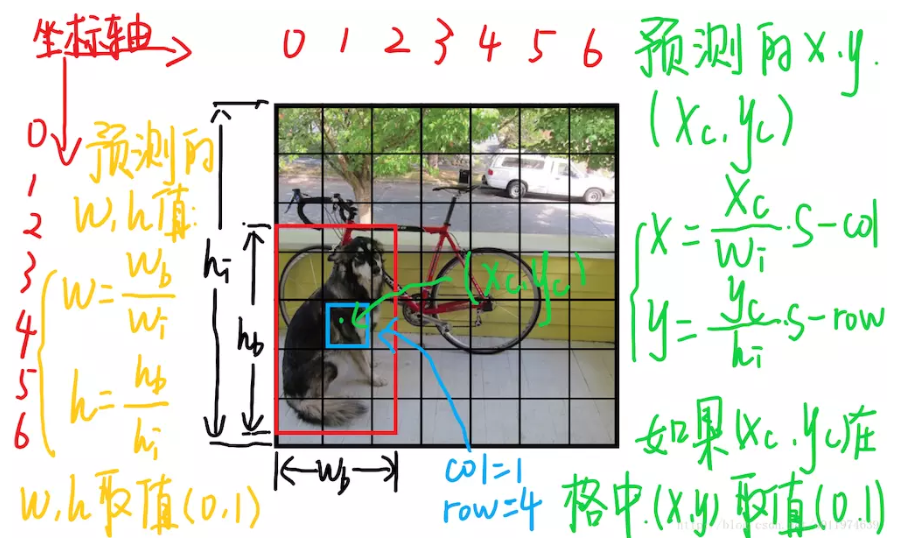# 前言

Faster R-CNNYOLOSSD在通用目标检测领域有着奠基一般的作用， 而YOLOv2YOLOv3由于其灵活易用的特性，在工业界一直很受欢迎，下面这篇文章主要想从损失函数的角度集中讨论下这几个主流框架的区别。

# 分类损失

• Faster R-CNN
需要注意的是，这里的Faster R-CNN讨论的是RPN的损失，所以在分类损失中，Faster R-CNN的RPN用的是二值交叉熵，因为RPN分类是区分当前的区域是不是个目标的二分类问题。
• SSD
SSD，是one-stage的结构，没有区域建议，它的分类损失是交叉熵，如果是针对VOC数据集，那么类别应该是20类，针对COCO数据集，类别是80类。
此外还有一个小区别是，SSD有背景类，比如COCO数据集上，SSD要区域81类。
• YOLO，YOLOv2
YOLO和YOLOv2是one-stage的结构，没有区域建议，但是YOLO和YOLOv2做分类的时候用的L2 loss，是将分类问题当作回归任务来处理。
• YOLOv3
YOLOv3和YOLOv2、SSD都不同，它的分类损失既不使用softmax+交叉熵来做，又没有用L2，而是使用n个二值交叉熵来做，比如在COCO上，使用一个80类的交叉熵是可以实现的，但是YOLOv3用了Logistic+二值交叉熵处理，将一个80分类问题转化为80个二分类问题。

# 回归损失

## 边界框的中心点

• Faster R-CNN和SSD
Faster R-CNN的RPN和SSD在处理边界框的中心点时采用的是相同的思路，并且它们都有Anchor，其实是SSD借鉴了RPN，最后一层特征图上的点决定了预设的中心点，RPN和SSD要预测的是Ground Truth对中心点的offset，并除Anchor的宽高后的结果，假设预设框为 { d c x , d c y , d w , d h } \left \{d^{cx},d^{cy},d^{w},d^{h}\right \} ，ground truth为 { g c x , g c y , g w , g h } \left \{g^{cx},g^{cy},g^{w},g^{h}\right \} ，从公式中可以看出来， { d c x , d c y } \left \{d^{cx},d^{cy}\right \} { g c x , g c y } \left \{g^{cx},g^{cy}\right \} 都表示中心点坐标。那么转换后的ground truth坐标 { g ^ c x , g ^ c y } \left \{\hat{g}^{cx},\hat{g}^{cy}\right \} 依照下面方式求取：

g ^ c x = ( g c x − d c x ) d w \hat{g}^{cx}=\frac{\left (g^{cx}-d^{cx} \right )}{d^{w}} g ^ c y = ( g c y − d c y ) d h \hat{g}^{cy}=\frac{\left (g^{cy}-d^{cy} \right )}{d^{h}}

• YOLO，YOLOv2和YOLOv3
YOLO系列的结构中，YOLO是没有Anchor的，YOLO只有格子，YOLOv2和YOLOv3带Anchor，但是这并不影响它们边界框中心点的选择，它们的边界框中心都是在预测距离格子左上角点的offset，这一点和Faster R-CNN与SSD是不同的。特别说明，上图来自《YOLO文章详细解读》
对于上图中蓝色框对应的格子(坐标为 ( x c o l = 1 , y r o w = 4 ) (x_{col}=1,y_{row}=4) )，假设它预测的输出是红色框的bbox,设bbox的中心坐标为 ( x c , y c ) (x_c,y_c) ,那么最终预测出来的 ( x , y ) (x,y) 是经过归一化处理的，表示的是中心相对于单元格的offset，计算公式如下。其中 k k 是格子的数量， w i m g w_{img} h i m g h_{img} 是图片的宽高。
x = x c w i m g k − x c o l x= \frac{x_{c}}{w_{img}}k-x_{col}
y = y c h i m g k − y r o w y= \frac{y_{c}}{h_{img}}k-y_{row}

## 边界框的尺寸

• YOLO
在边界框尺寸这个方面，YOLO显得非常孤立，因为它是这5个结构中唯一无Anchor的，由于没有先验，所以YOLO在表达 ( w , h ) (w,h) 时非常的简单粗暴，就是相对于整个图片的比例做了归一化。
w = w b w i m g w= \frac{w_{b}}{w_{img}} h = h b h i m g h= \frac{h_{b}}{h_{img}}特别说明，上图来自《YOLO文章详细解读》

• Faster R-CNN，SSD，YOLOv2和YOLOv3
Faster R-CNN，SSD，YOLOv2和YOLOv3都带Anchor，所以它们对于 ( w , h ) (w,h) 的处理是一致的，就是根据Anchor，用对数函数，对预测值和ground truth去重新编码。
下面是SSD中用Anchor编码的ground truth例子：假设预设框为 { d c x , d c y , d w , d h } \left \{d^{cx},d^{cy},d^{w},d^{h}\right \} ，ground truth为 { g c x , g c y , g w , g h } \left \{g^{cx},g^{cy},g^{w},g^{h}\right \} ，从公式中可以看出来。那么转换后的ground truth坐标 { g ^ w , g ^ h } \left \{\hat{g}^{w},\hat{g}^{h}\right \} 依照下面方式求取：
g ^ w = l o g g w d w \hat{g}^{w}=log{\frac{g_{w}}{d^{w}}} g ^ h = l o g g h d h \hat{g}^{h}=log{\frac{g_{h}}{d^{h}}}
这里使用的是 l o g log ，其实可以看做 l n ln
在YOLOv2中这个形式会变一下，但是本质上是一样的，总结来看，根据边界框预测的4个offset { t x , t y , t w , t h } \left \{ t_{x}, t_{y},t_{w},t_{h} \right \} ，可以按照下列公式计算出ground truth在特征图上的相对值 { b x , b y , b w , b h } \left \{ b_{x}, b_{y},b_{w},b_{h} \right \}
b w = p w e t w b_{w}= p_{w}e^{t_{w}} b h = p h e t h b_{h}= p_{h}e^{t_{h}}
上式中把 p w p_{w} 除过来，然后两边取对数，就和SSD一样了。
所以这就是为什么Faster R-CNN，SSD，YOLOv2和YOLOv3中都有log，就是为了根据Anchor对预测值和ground truth去重新编码。

## 损失函数

• Faster R-CNN和SSD
SSD可以说在边界框回归问题上完全参考RPN，包括损失函数，所以它们都用smooth L1损失。
• YOLO，YOLOv2和YOLOv3
YOLO系列在边界框回归损失上用的是L2，并没有参考RPN。

# 其他损失

• Faster R-CNN和SSD
PRN和SSD是没有其他损失函数的，因为RPN只有两类，其中包含了负类，SSD的类别中也包含背景类。
• YOLO，YOLOv2和YOLOv3
YOLO系列在最后的类别输出上是不包含背景类的，所以它在输出上加上了一个confidence，所以YOLO系列处理基础的分类损失和回归损失外，还需要有一个confidence损失，去评价当前的区域是object还是no object。01-141681
05-282325
09-277840
09-181万+
03-08292
03-301万+
05-29846
11-133717
11-082948
©️2020 CSDN 皮肤主题: Age of Ai 设计师:meimeiellie